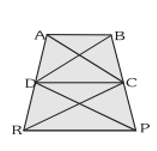Most Affordable JEE | NEET | 8,9,10 Preparation by Kota's Top IITian Doctor Faculties

# NCERT Solutions for Class 9 Maths chapter 9 Exercise 9.3 - Areas of Parallelograms and Triangles

Class 9Hey, are you a class 9 Student and Looking for Ways to Download NCERT Solutions for Class 9 Maths chapter 9 Exercise 9.3? If Yes then you are at the right place.

Here we have listed Class 9 maths chapter 9 exercise 9.3 solutions in PDF that is prepared by Kota’s top IITian’s Faculties by keeping Simplicity in mind.

If you want to score high in your class 9 Maths Exam then it is very important for you to have a good knowledge of all the important topics, so to learn and practice those topics you can use eSaral NCERT Solutions.

So, without wasting more time Let’s start.

### Download The PDF of NCERT Solutions for Class 9 Maths chapter 9 Exercise 9.3 "Areas of Parallelograms and Triangles"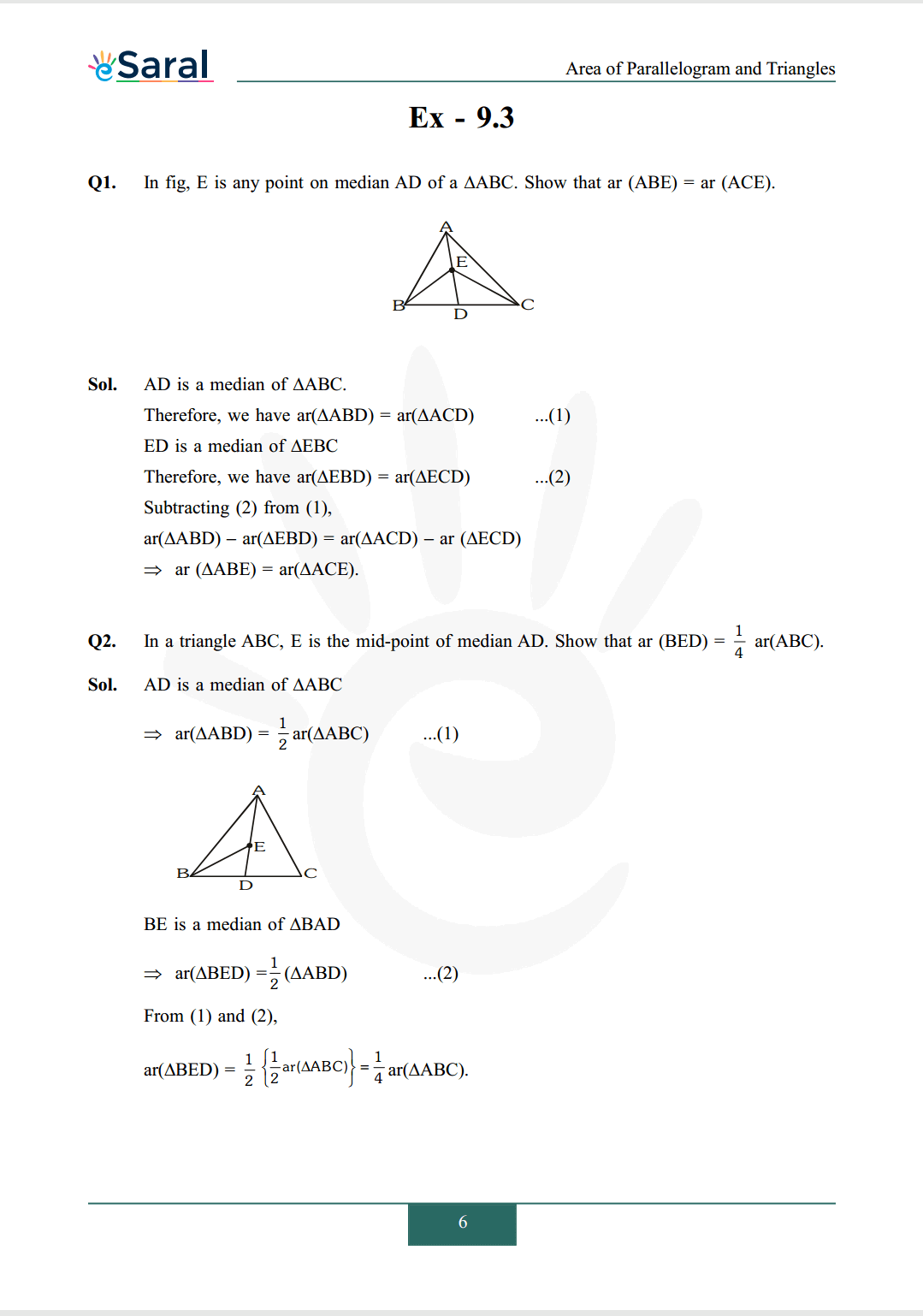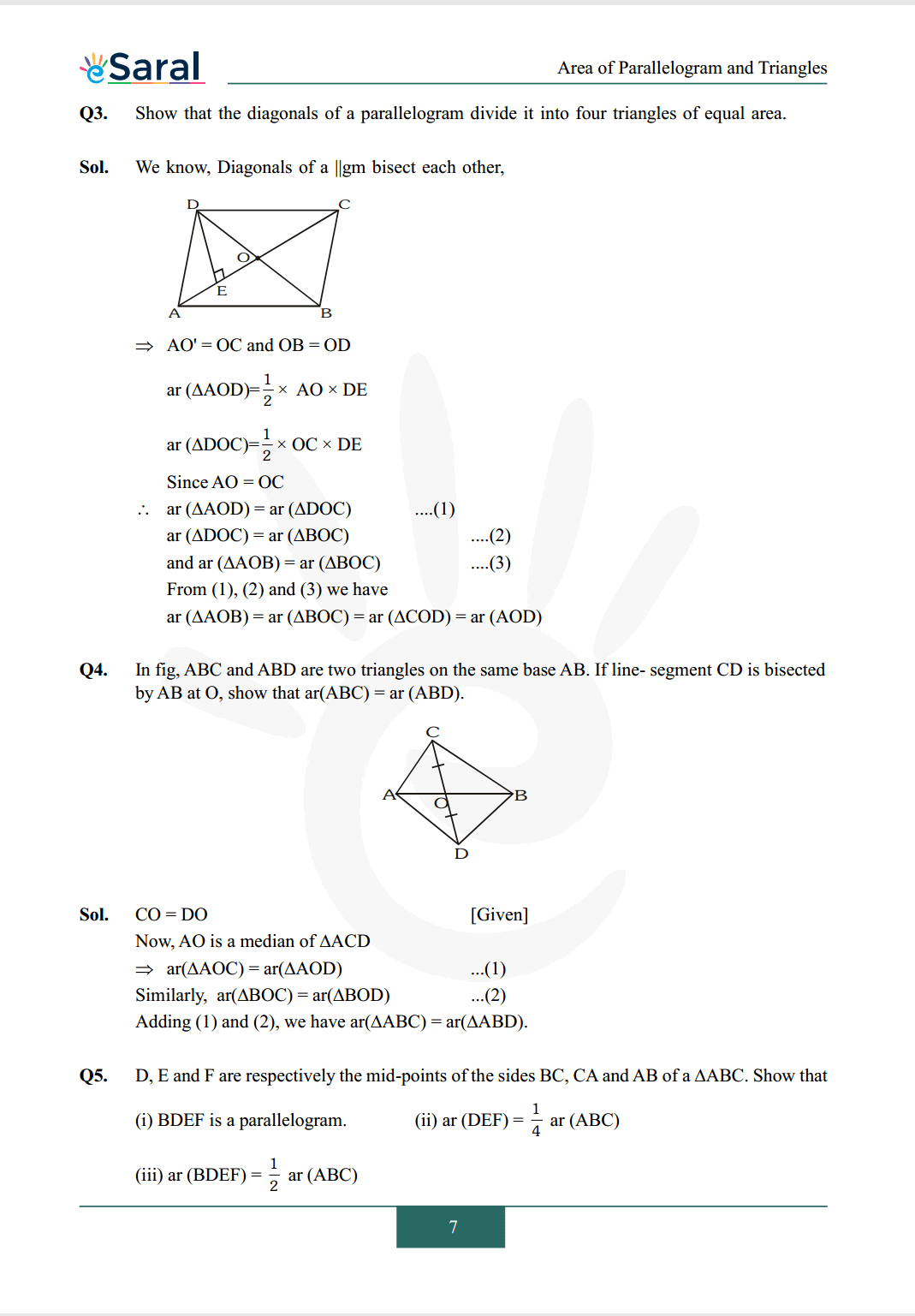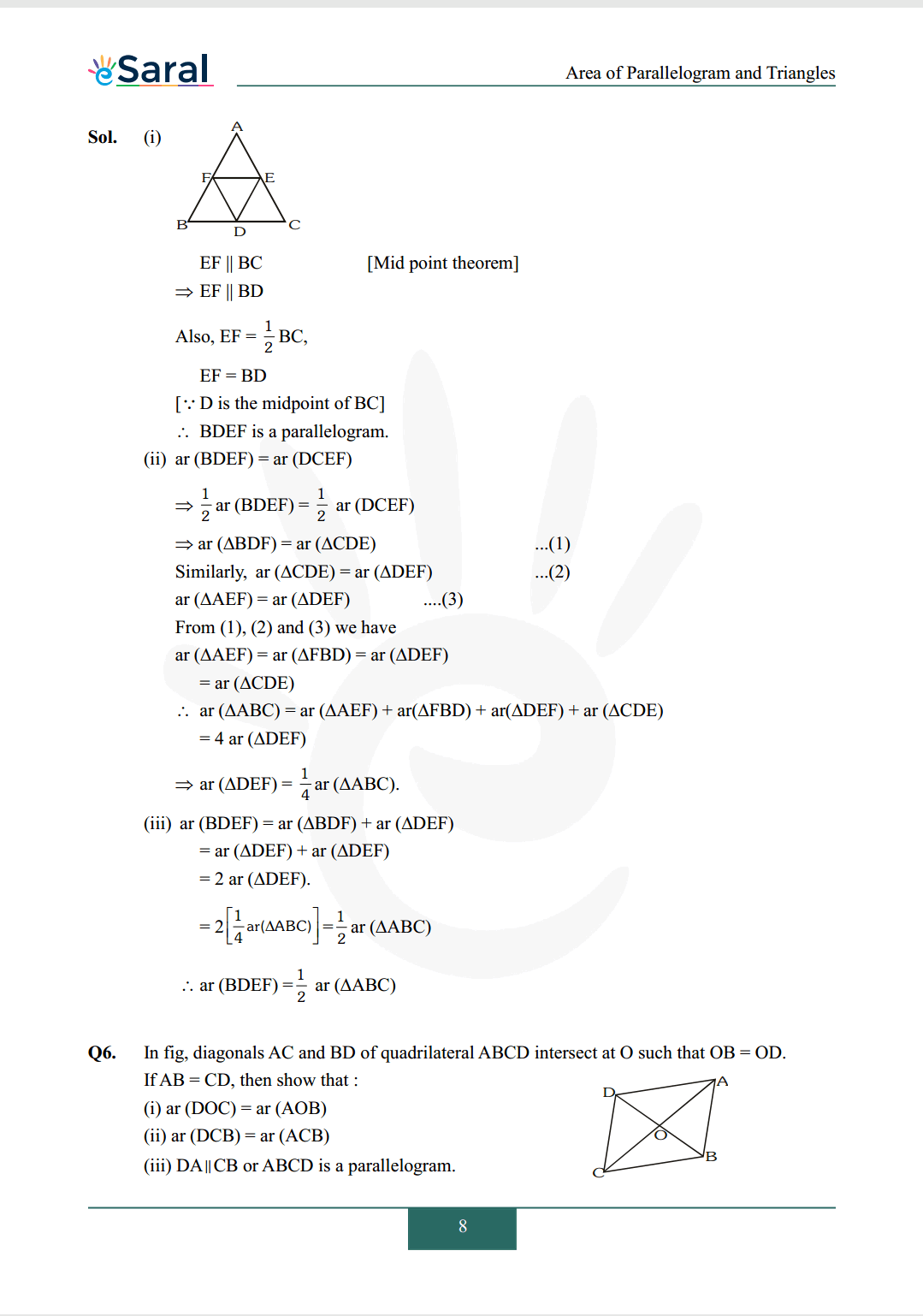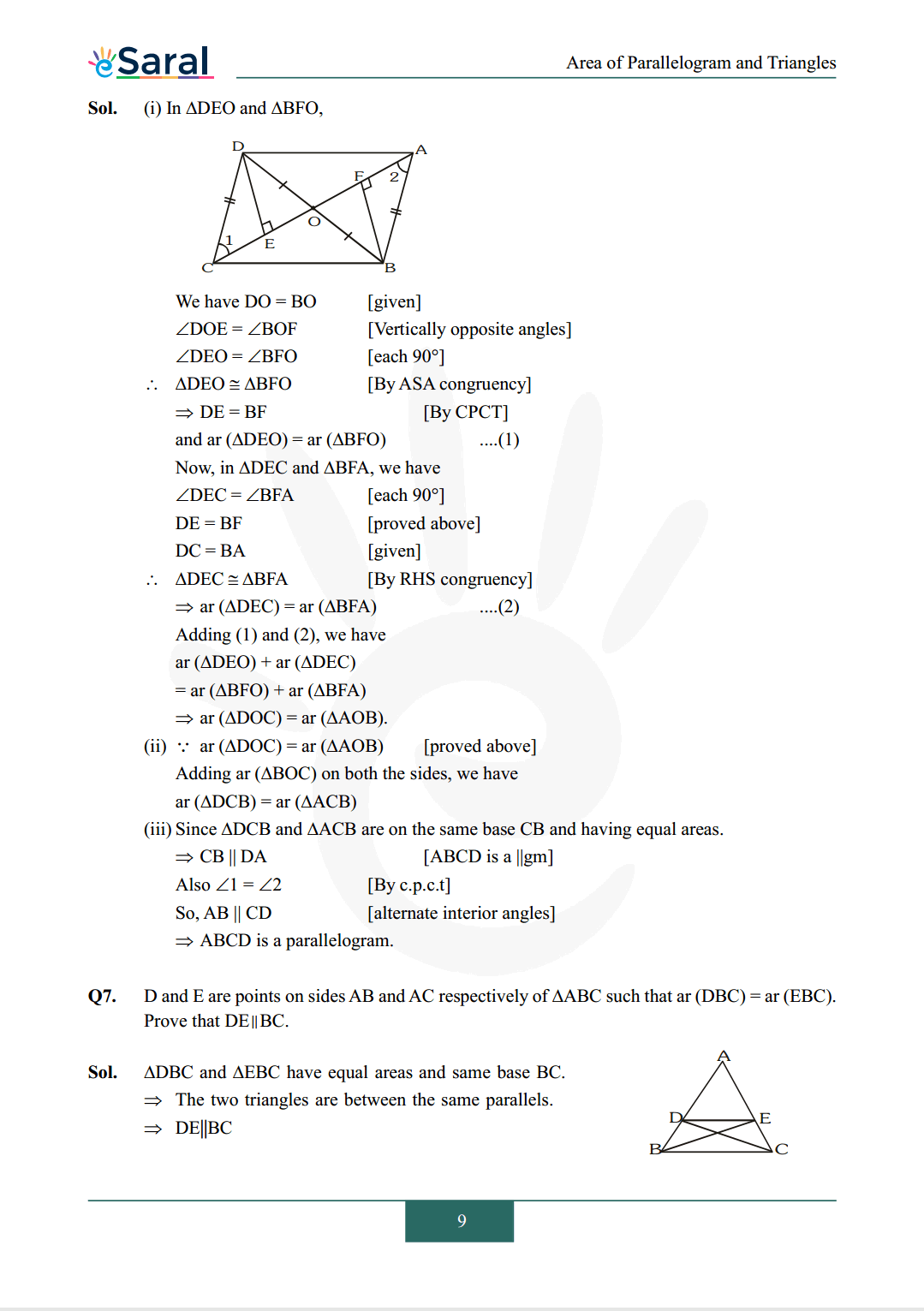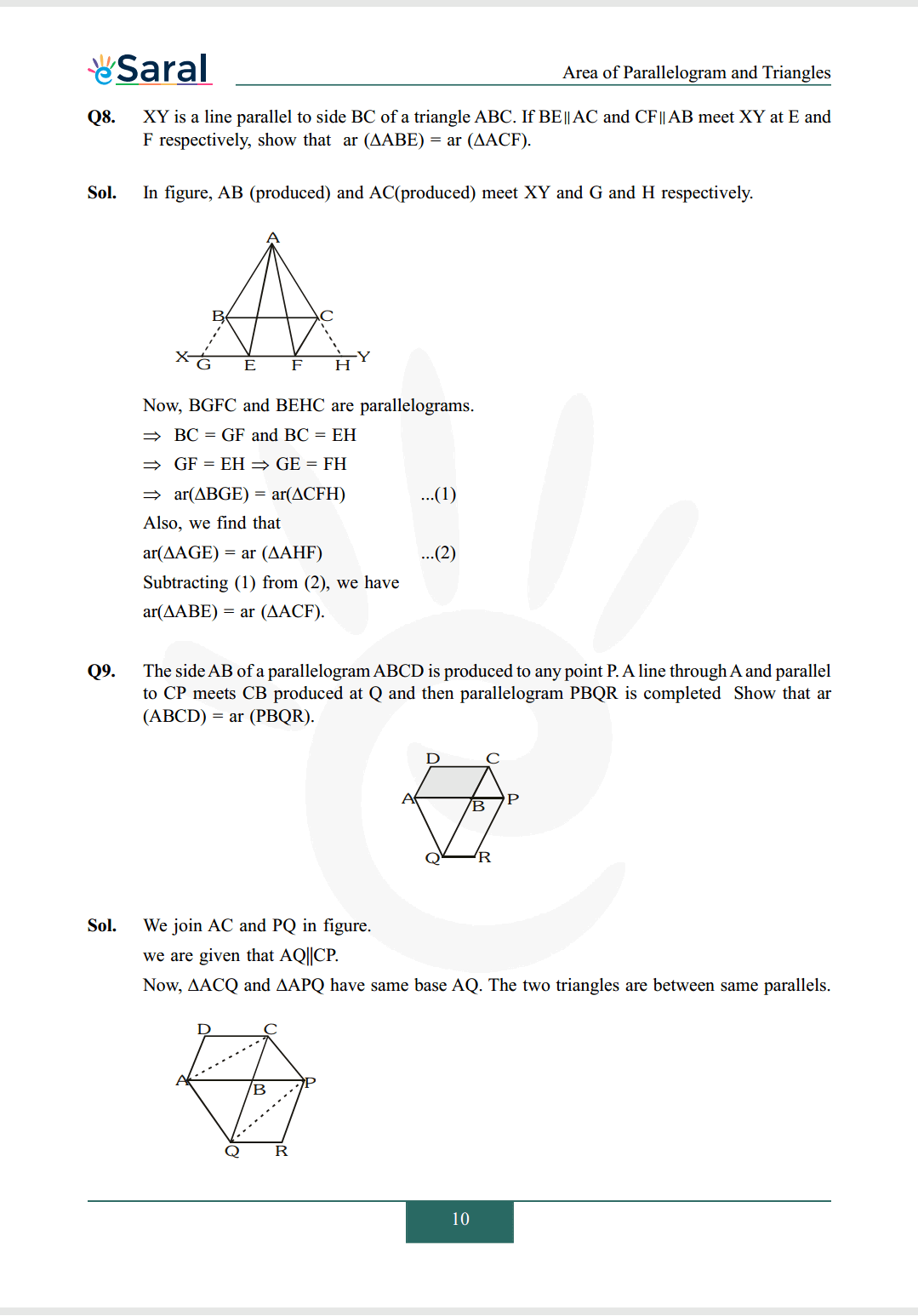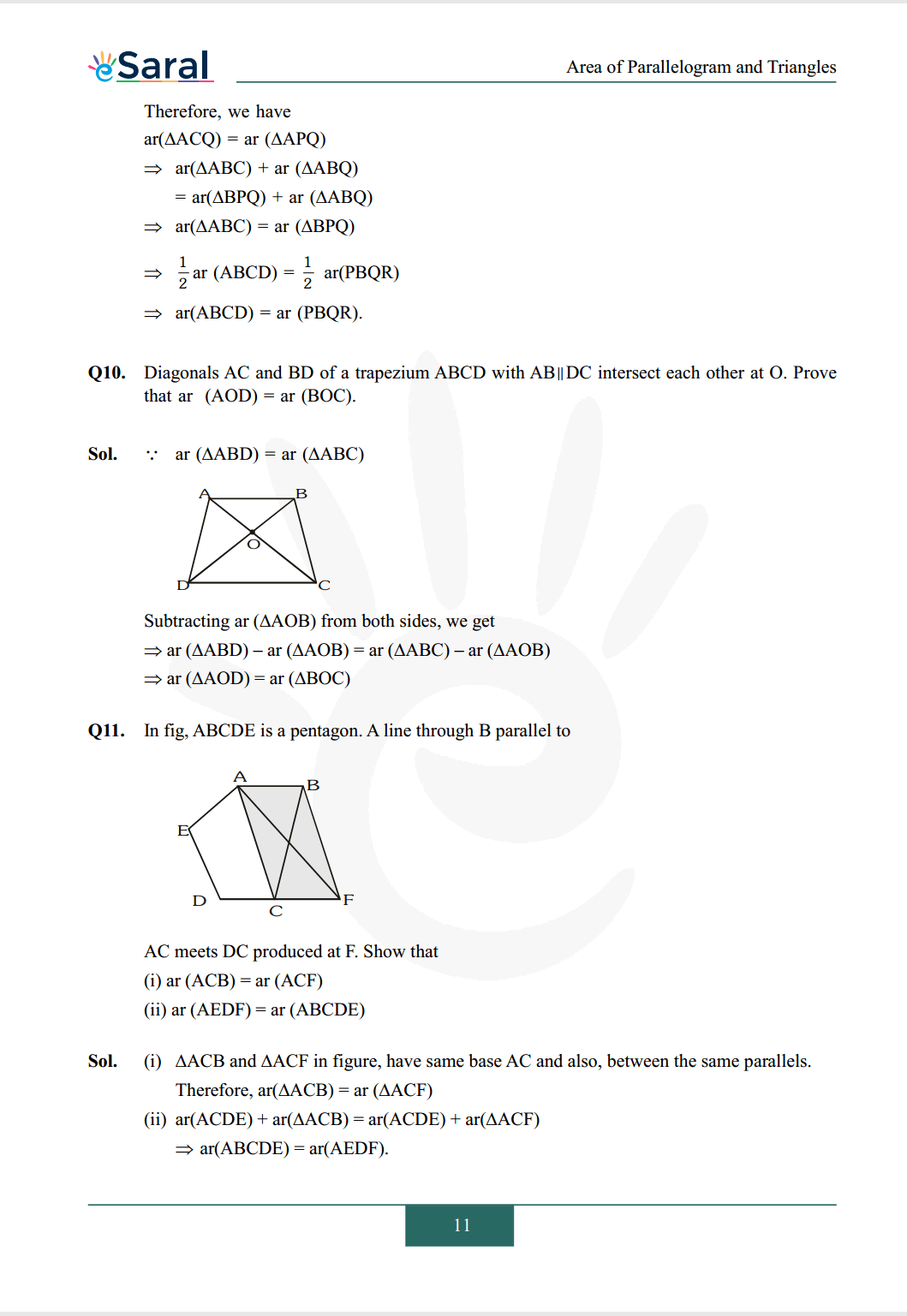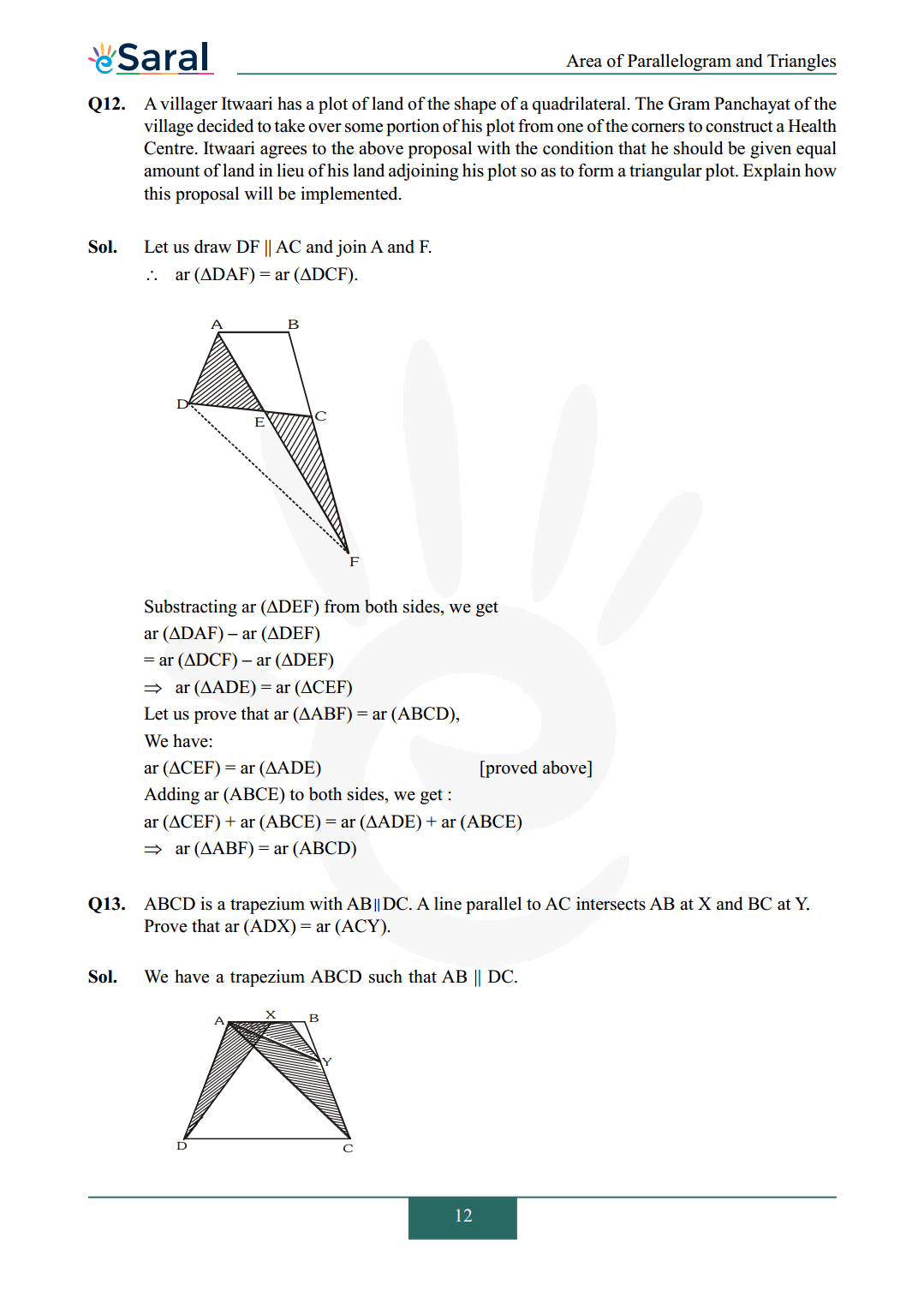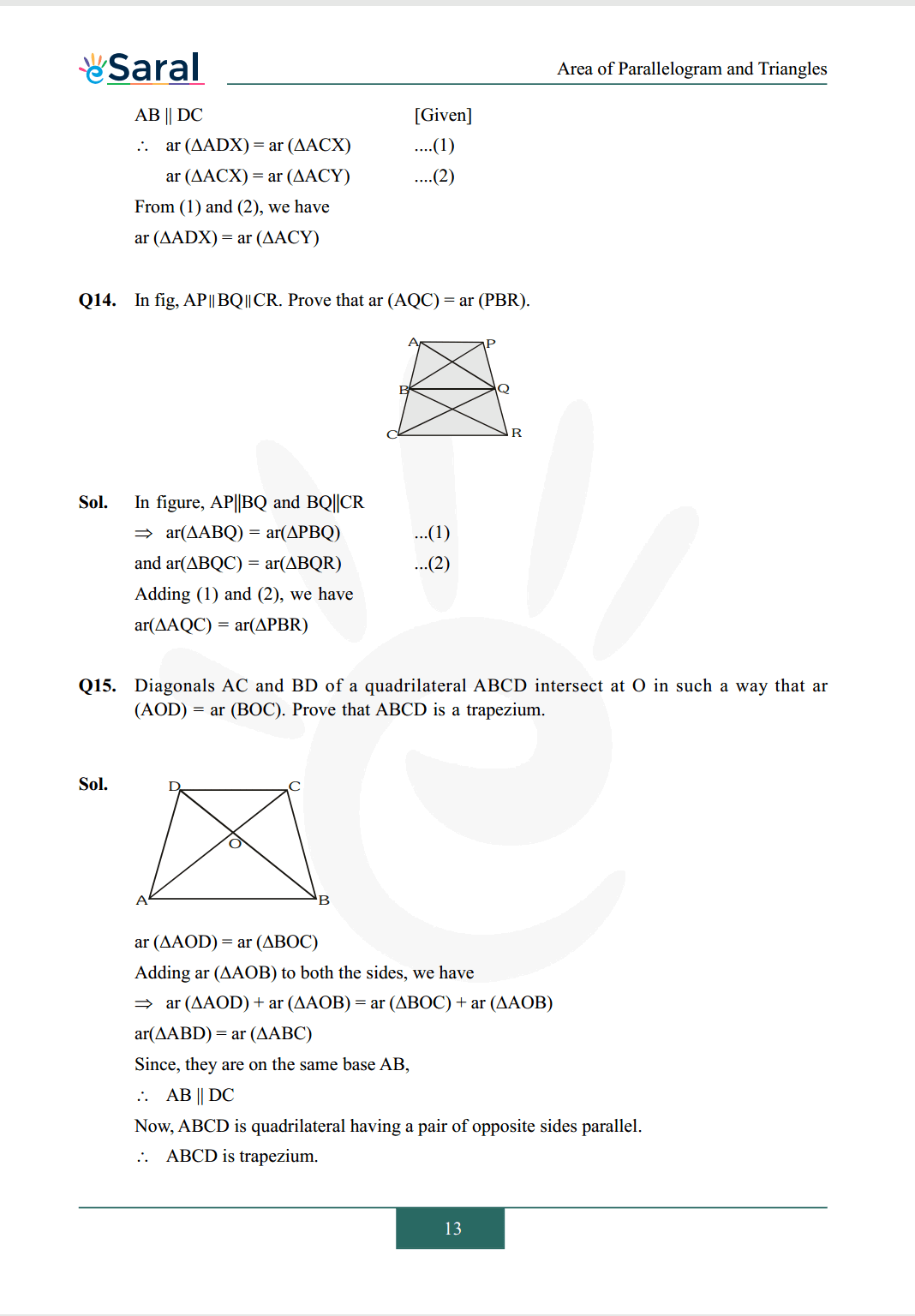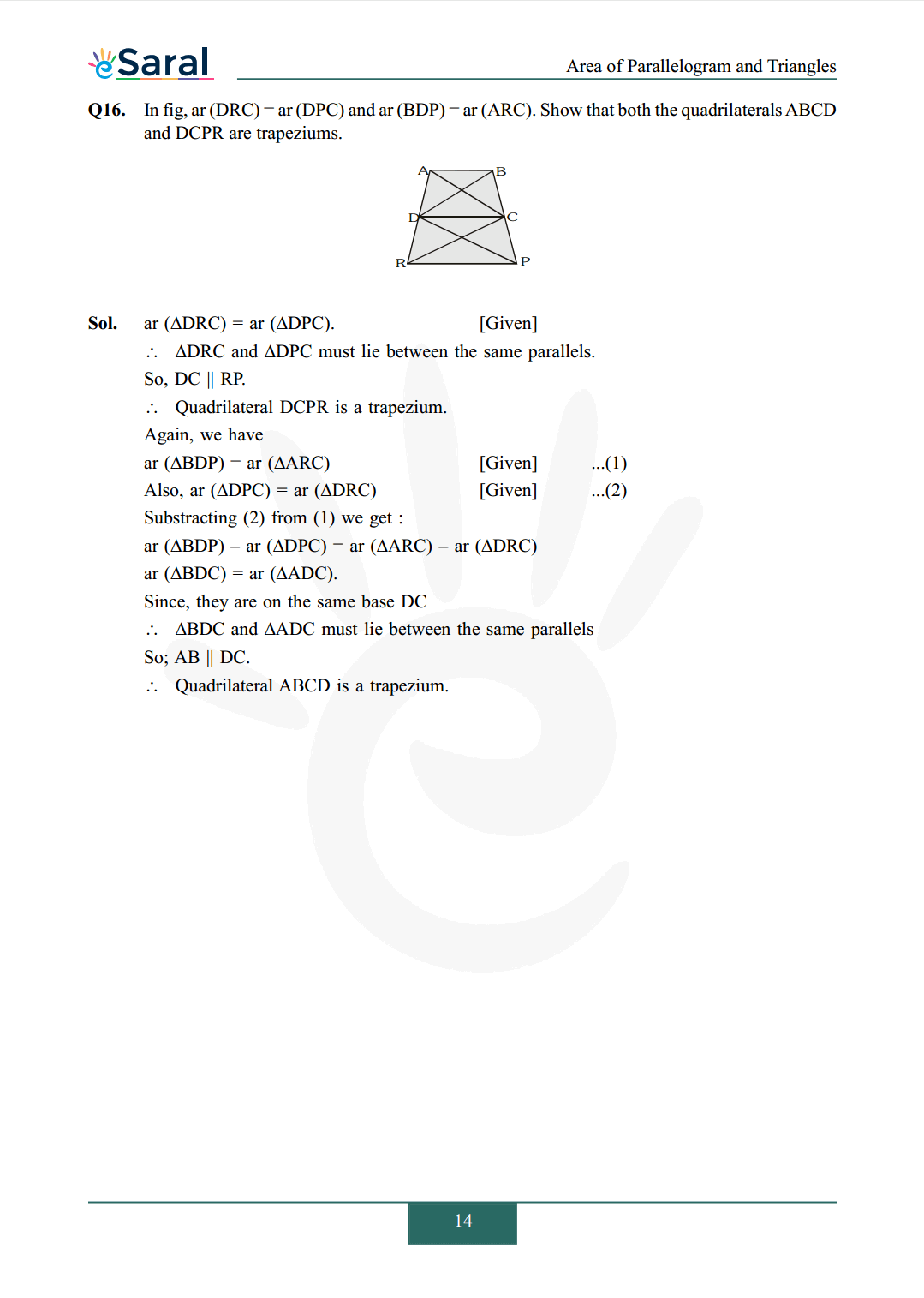#### All Questions of Chapter 9 Exercise 9.3

Once you complete the chapter 9 then you can revise Ex. 9.3 by solving following questions

Q1. In fig, E is any point on median AD of a $\Delta \mathrm{ABC}$. Show that ar $(\mathrm{ABE})=\operatorname{ar}(\mathrm{ACE})$.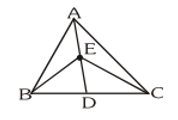Q2. In a triangle $\mathrm{ABC}, \mathrm{E}$ is the mid-point of median $\mathrm{AD}$. Show that ar $(\mathrm{BED})=\frac{1}{4} \operatorname{ar}(\mathrm{ABC})$.

Q3. Show that the diagonals of a parallelogram divide it into four triangles of equal area.

Q4. In fig, $\mathrm{ABC}$ and $\mathrm{ABD}$ are two triangles on the same base $\mathrm{AB}$. If line- segment $\mathrm{CD}$ is bisected by $\mathrm{AB}$ at $\mathrm{O}$, show that $\operatorname{ar}(\mathrm{ABC})=\operatorname{ar}(\mathrm{ABD})$.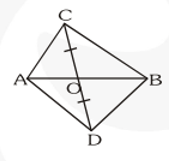Q5. D, E and $\mathrm{F}$ are respectively the mid-points of the sides $\mathrm{BC}, \mathrm{CA}$ and $\mathrm{AB}$ of $\mathrm{a} \triangle \mathrm{ABC}$. Show that
(i) $\mathrm{BDEF}$ is a parallelogram.
(ii) $\operatorname{ar}(\mathrm{DEF})=\frac{1}{4} \operatorname{ar}(\mathrm{ABC})$
(iii) ar $(\mathrm{BDEF})=\frac{1}{2}$ ar $(\mathrm{ABC})$

Q6. In fig, diagonals AC and BD of quadrilateral ABCD intersect at $\mathrm{O}$ such that $\mathrm{OB}=\mathrm{OD}$. If $\mathrm{AB}=\mathrm{CD}$, then show that :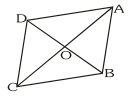(i) $\operatorname{ar}(\mathrm{DOC})=\operatorname{ar}(\mathrm{AOB})$
(ii) $\operatorname{ar}(\mathrm{DCB})=\operatorname{ar}(\mathrm{ACB})$
(iii) $\mathrm{DA} \| \mathrm{CB}$ or $\mathrm{ABCD}$ is a parallelogram.

Q7. $\mathrm{D}$ and $\mathrm{E}$ are points on sides $\mathrm{AB}$ and $\mathrm{AC}$ respectively of $\Delta \mathrm{ABC}$ such that $\operatorname{ar}(\mathrm{DBC})=\operatorname{ar}(\mathrm{EBC})$. Prove that $\mathrm{DE} \| \mathrm{BC}$.

Q8. $\mathrm{XY}$ is a line parallel to side $\mathrm{BC}$ of a triangle $\mathrm{ABC}$. If $\mathrm{BE} \| \mathrm{AC}$ and $\mathrm{CF} \| \mathrm{AB}$ meet $\mathrm{XY}$ at $\mathrm{E}$ and $F$ respectively, show that ar $(\Delta \mathrm{ABE})=$ ar $(\Delta \mathrm{ACF})$.

Q9. The side $A B$ of a parallelogram $A B C D$ is produced to any point $P$. A line through A and parallel to CP meets CB produced at $\mathrm{Q}$ and then parallelogram $\mathrm{PBQR}$ is completed Show that ar $(\mathrm{ABCD})=\operatorname{ar}(\mathrm{PBQR}) .$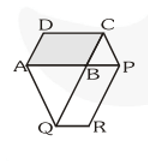Q10. Diagonals AC and BD of a trapezium $\mathrm{ABCD}$ with $\mathrm{AB} \| \mathrm{DC}$ intersect each other at $\mathrm{O}$. Prove that ar $(\mathrm{AOD})=\operatorname{ar}(\mathrm{BOC})$.

Q11. In fig, $\mathrm{ABCDE}$ is a pentagon. A line through B parallel to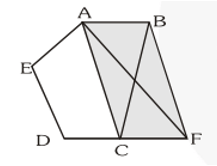AC meets DC produced at $\mathrm{F}$. Show that
(i) $\operatorname{ar}(\mathrm{ACB})=\operatorname{ar}(\mathrm{ACF})$
(ii) $\operatorname{ar}(\mathrm{AEDF})=\operatorname{ar}(\mathrm{ABCDE})$

Q12. A villager Itwaari has a plot of land of the shape of a quadrilateral. The Gram Panchayat of the village decided to take over some portion of his plot from one of the corners to construct a Health Centre. Itwaari agrees to the above proposal with the condition that he should be given equal amount of land in lieu of his land adjoining his plot so as to form a triangular plot. Explain how this proposal will be implemented.

Q13. $\mathrm{ABCD}$ is a trapezium with $\mathrm{AB} \| \mathrm{DC}$. A line parallel to $\mathrm{AC}$ intersects $\mathrm{AB}$ at $\mathrm{X}$ and $\mathrm{BC}$ at $\mathrm{Y}$. Prove that ar $(\mathrm{ADX})=\operatorname{ar}(\mathrm{ACY})$.

Q14. In fig, $\mathrm{AP}\|\mathrm{BQ}\| \mathrm{CR}$. Prove that ar $(\mathrm{AQC})=\operatorname{ar}(\mathrm{PBR})$.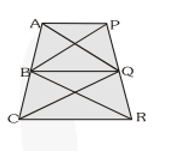Q15. Diagonals $\mathrm{AC}$ and $\mathrm{BD}$ of a quadrilateral $\mathrm{ABCD}$ intersect at $\mathrm{O}$ in such a way that ar $(\mathrm{AOD})=\operatorname{ar}(\mathrm{BOC})$. Prove that $\mathrm{ABCD}$ is a trapezium.

Q16. In fig, ar $(\mathrm{DRC})=\operatorname{ar}(\mathrm{DPC})$ and ar $(\mathrm{BDP})=\operatorname{ar}(\mathrm{ARC})$. Show that both the quadrilaterals $\mathrm{ABCD}$ and DCPR are trapeziums.